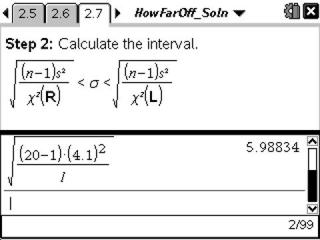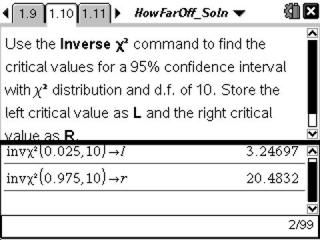# Activities

••• ##### Subject Area

• Math: Statistics: Inferential Statistics

• ##### Author9-12

15 Minutes

• ##### Device
• TI-Nspire™ Navigator™
• TI-Nspire™
• TI-Nspire™ CAS
• ##### Software

TI-Nspire™
TI-Nspire™ CAS

2.0

• ##### Report an Issue

How Far Am I Off?#### Activity Overview

Students calculate a confidence interval using the chi-square distribution to estimate a population variance.

#### Key Steps

•Students review the chi-square distribution and discuss how the non-symmetric distribution requires them to find two critical values when calculating a confidence interval that estimates the population variance.

•Then students are to calculate the 95% confidence interval for the population variance given the standard deviation of a random sample.